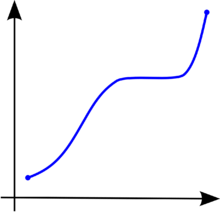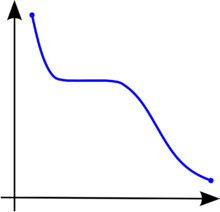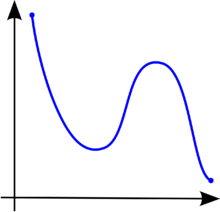# Monotonic function

"Monotonicity" redirects here. For information on monotonicity as it pertains to voting systems, see monotonicity criterion.
"Monotonic" redirects here. For other uses, see Monotone (disambiguation).Figure 1. A monotonically increasing function. It is strictly increasing on the left and right while just non-decreasing in the middle.Figure 2. A monotonically decreasing functionFigure 3. A function that is not monotonic

In mathematics, a monotonic function (or monotone function) is a function between ordered sets that preserves or reverses the given order. This concept first arose in calculus, and was later generalized to the more abstract setting of order theory.

## Monotonicity in calculus and analysis

In calculus, a functiondefined on a subset of the real numbers with real values is called monotonic if and only if it is either entirely increasing or decreasing. It is called monotonically increasing (also increasing or nondecreasing), if for allandsuch thatone has, sopreserves the order (see Figure 1). Likewise, a function is called monotonically decreasing (also decreasing or nonincreasing) if, whenever, then, so it reverses the order (see Figure 2).

If the orderin the definition of monotonicity is replaced by the strict order, then one obtains a stronger requirement. A function with this property is called strictly increasing. Again, by inverting the order symbol, one finds a corresponding concept called strictly decreasing. Functions that are strictly increasing or decreasing are one-to-one (because fornot equal to, eitherorand so, by monotonicity, eitheror, thusis not equal to.)

When functions between discrete sets are considered in combinatorics, it is not always obvious that "increasing" and "decreasing" are taken to include the possibility of repeating the same value at successive arguments, so one finds the terms weakly increasing and weakly decreasing to stress this possibility.

The terms "non-decreasing" and "non-increasing" should not be confused with the (much weaker) negative qualifications "not decreasing" and "not increasing". For example, the function of figure 3 first falls, then rises, then falls again. It is therefore not decreasing and not increasing, but it is neither non-decreasing nor non-increasing.

The term monotonic transformation can also possibly cause some confusion because it refers to a transformation by a strictly increasing function. Notably, this is the case in economics with respect to the ordinal properties of a utility function being preserved across a monotonic transform (see also monotone preferences).

A functionis said to be absolutely monotonic over an intervalif the derivatives of all orders ofare nonnegative or all nonpositive at all points on the interval.

### Some basic applications and results

The following properties are true for a monotonic function:

•has limits from the right and from the left at every point of its domain;
•has a limit at positive or negative infinity () of either a real number,, or.
•can only have jump discontinuities;
•can only have countably many discontinuities in its domain. The discontinuities, however, do not necessarily consist of isolated points and may even be dense in an interval (a,b).

These properties are the reason why monotonic functions are useful in technical work in analysis. Two facts about these functions are:

• ifis a monotonic function defined on an interval, thenis differentiable almost everywhere on, i.e. the setof numbersinsuch thatis not differentiable inhas Lebesgue measure zero. In addition, this result cannot be improved to countable: see Cantor function.
• ifis a monotonic function defined on an interval, thenis Riemann integrable.

An important application of monotonic functions is in probability theory. Ifis a random variable, its cumulative distribution functionis a monotonically increasing function.

A function is unimodal if it is monotonically increasing up to some point (the mode) and then monotonically decreasing.

Whenis a strictly monotonic function, thenis injective on its domain, and ifis the range of, then there is an inverse function onfor.

## Monotonicity in topology

A map f : X  Y is said to be monotone if each of its fibers is connected i.e. for each element y in Y the (possibly empty) set f−1(y) is connected.

## Monotonicity in functional analysis

In functional analysis on a topological vector space X, a (possibly non-linear) operator T : X  X is said to be a monotone operator ifKachurovskii's theorem shows that convex functions on Banach spaces have monotonic operators as their derivatives.

A subset G of X × X is said to be a monotone set if for every pair [u1,w1] and [u2,w2] in G,G is said to be maximal monotone if it is maximal among all monotone sets in the sense of set inclusion. The graph of a monotone operator G(T) is a monotone set. A monotone operator is said to be maximal monotone if its graph is a maximal monotone set.

## Monotonicity in order theory

Order theory deals with arbitrary partially ordered sets and preordered sets in addition to real numbers. The above definition of monotonicity is relevant in these cases as well. However, the terms "increasing" and "decreasing" are avoided, since their conventional pictorial representation does not apply to orders that are not total. Furthermore, the strict relations < and > are of little use in many non-total orders and hence no additional terminology is introduced for them.

A monotone function is also called isotone, or order-preserving. The dual notion is often called antitone, anti-monotone, or order-reversing. Hence, an antitone function f satisfies the property

xy implies f(x) ≥ f(y),

for all x and y in its domain. The composite of two monotone mappings is also monotone.

A constant function is both monotone and antitone; conversely, if f is both monotone and antitone, and if the domain of f is a lattice, then f must be constant.

Monotone functions are central in order theory. They appear in most articles on the subject and examples from special applications are found in these places. Some notable special monotone functions are order embeddings (functions for which xy if and only if f(x) ≤ f(y)) and order isomorphisms (surjective order embeddings).

## Monotonicity in the context of search algorithms

In the context of search algorithms monotonicity (also called consistency) is a condition applied to heuristic functions. A heuristic h(n) is monotonic if, for every node n and every successor n' of n generated by any action a, the estimated cost of reaching the goal from n is no greater than the step cost of getting to n' plus the estimated cost of reaching the goal from n' ,This is a form of triangle inequality, with n, n', and the goal Gn closest to n. Because every monotonic heuristic is also admissible, monotonicity is a stricter requirement than admissibility. In some heuristic algorithms, such as A*, the algorithm can be considered optimal if it is monotonic.

## Boolean functions

In Boolean algebra, a monotonic function is one such that for all ai and bi in {0,1}, if a1b1, a2b2, ..., anbn (i.e. the Cartesian product {0, 1}n is ordered coordinatewise), then f(a1, ..., an) ≤ f(b1, ..., bn). In other words, a Boolean function is monotonic if, for every combination of inputs, switching one of the inputs from false to true can only cause the output to switch from false to true and not from true to false. Graphically, this means that a Boolean function is monotonic when in its Hasse diagram (dual of its Venn diagram), there is no 1 connected to a higher 0.

The monotonic Boolean functions are precisely those that can be defined by an expression combining the inputs (which may appear more than once) using only the operators and and or (in particular not is forbidden). For instance "at least two of a,b,c hold" is a monotonic function of a,b,c, since it can be written for instance as ((a and b) or (a and c) or (b and c)).

The number of such functions on n variables is known as the Dedekind number of n.

1. See the section on Cardinal Versus Ordinal Utility in Simon & Blume (1994).
2. Conditions for optimality: Admissibility and consistency pg. 94-95 (Russell & Norvig 2010).

## Bibliography

• Bartle, Robert G. (1976). The elements of real analysis (second ed.).
• Grätzer, George (1971). Lattice theory: first concepts and distributive lattices. ISBN 0-7167-0442-0.
• Pemberton, Malcolm; Rau, Nicholas (2001). Mathematics for economists: an introductory textbook. Manchester University Press. ISBN 0-7190-3341-1.
• Renardy, Michael & Rogers, Robert C. (2004). An introduction to partial differential equations. Texts in Applied Mathematics 13 (Second ed.). New York: Springer-Verlag. p. 356. ISBN 0-387-00444-0.
• Riesz, Frigyes & Béla Szőkefalvi-Nagy (1990). Functional Analysis. Courier Dover Publications. ISBN 978-0-486-66289-3.
• Russell, Stuart J.; Norvig, Peter (2010). Artificial Intelligence: A Modern Approach (3rd ed.). Upper Saddle River, New Jersey: Prentice Hall. ISBN 978-0-13-604259-4.
• Simon, Carl P.; Blume, Lawrence (April 1994). Mathematics for Economists (first ed.). ISBN 978-0-393-95733-4. (Definition 9.31)